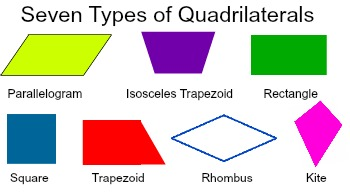03.28.19

There Are Two Sides to Every Story. And for a Lot of Them, There Are Four

This month, things are shaping up as we look at those polygons of virtue, the quadrilaterals. We’ll delve into their humble beginnings, the different types, their inclusion and influence in our culture as well as in branding and corporate marketing.

From the Latin quadri (“four”) and latus (“side”), a quadrilateral is a rather plain plane with four sides and four angles. It’s also called a tetragon, or a polygon (a closed figure with three or more usually straight sides). But for our purposes, let’s stick with quads and not muddy the waters.

Greek math geek and philosopher Pythagoras is credited with the theorem that states “the area of the square build upon the hypotenuse of a right triangle is equal to the sum of the areas of the squares upon the remaining sides.” In other words, he defined the quadrilateral. Legend has it he was so pleased with his new theory, he made the humble gesture of sacrificing some oxen.

After this discovery, a new list of quads was added to the world’s list of shapes. But it wasn’t until 1813 that English mathematician J.P. Smith came up with the trapezium (a quadrilateral with no two sides parallel).

Now would be a good time to show and explain the seven types of quadrilaterals, which are:

• Square (a rectangle where all four side are of equal length).
• Rectangle (a parallelogram containing four right angles).
• Parallelogram (a quadrilateral where both pairs of opposite sides are parallel to each other).
• Rhombus (also called the equilateral quadrilateral, it has four sides all the same length…but while all squares are technically rhombuses not all rhombuses are squares. Stay with us…).
• Trapezoid (a quadrilateral which has two parallel and two non-parallel sides).
• Isosceles Trapezoid (a trapezoid where the two non-parallel sides are the same length).
• Kite (which contains two pairs of equal-length sides adjacent to each other…or more simply put, in the shape of a kite).And finally, quadrilaterals can be either convex or concave. If its interior angle is greater than 180 degrees, it’s concave. The best way to remember is that if it looks boxy it’s convex. If it looks like a boomerang, it’s concave.

I want more news